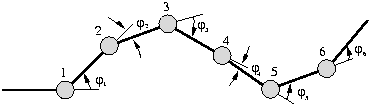## 1 Gait control tables

Each articulation is characterized by the angle between the two segments it links. The shape of the worm, at a given instant, is determined by the angular position vector. Figureshows a six articulations worm-like robot and the angular position vector at a given time.For every instant, an angular position vector there exist, determining the shape of the worm:. The control table is a matrix, which rows contains the angular position vectors for every instant. In order to generate the movement, the controller has to read the table, row by row, positioning the servos.

In robots like Polybot, this tables are pre-calculated and downloaded into the modules. Each table represents one gait. It is not possible to calculate or store all the possible tables for all the different gaits. Those tables are generated automatically in Cube.

Juan Gonzalez 2004-10-08